Disclosure: This post may contain affiliate links, meaning when you click the links and make a purchase, we receive a commission.

# How to Use FV, PV and PDURATION Functions in Excel

The PV Function, FV Function, and PDURATION Function are all extremely useful financial functions.

## Excel PV Function Syntax

The Present Value (PV) Function is used to calculate the present value of a loan or an investment using a constant interest rate. This is basically the amount at the beginning of the loan, or investment’s lifespan.

The syntax of the PV Function is:

=PV (rate, nper, pmt, [fv], [type])

– where rate is a required value and refers to the interest rate per period.

-nper is a required value and refers to the total number of payment periods.

-pmt is a required value and refers to the actual payment made each period. This is a constant amount over the duration of the investment/loan and cannot change.

-fv is an optional value and refers to a future value or balance that you want to have in your bank, after the last payment is made. If the fv is not included, Excel assumes fv is 0.

-type is an optional value that denotes when a payment is actually made. If the type argument is 0, this denotes that payment is made at the end of the period, whereas if the type argument is 1, this denotes that payment is made at the beginning of the period. If the type value is left out, Excel assumes that it is 0.

Read More: Financial Planning with Excel Solver [2 Case Studies]

A handy tip to remember when using financial functions and trying to figure out whether a number should be positive or negative is: if its money being paid by you, for example payment of a loan, then this number should be negative, whereas if its money you are receiving for example the returns from an investment you made, then the number should be positive.

## Excel FV Function Syntax

The Future Value (FV) Function is used to calculate the value of an investment or loan at some time in the future, based on a constant interest rate.

The syntax of the FV function is:

=FV (rate, nper, pmt, [pv], [type])

-where rate is a required value and refers to the interest rate per period.

-nper is a required value and refers to the total number of payment periods.

-pmt is a required value and refers to the actual payment made each period.

-pv is optional and refers to the present value that an investment is worth. If PV is omitted, Excel assumes that it is 0.

-type is an optional value that denotes when a payment is actually made. If the type argument is 0, this denotes that payment is made at the end of the period, whereas if the type argument is 1, this denotes that payment is made at the beginning of the period. If the type value is left out, Excel assumes that it is 0.

Read More: How to Use Compound Interest Formula in Excel

## Excel PDURATION Function Syntax

The PDURATION Function is a new financial function that was introduced in Excel 2013 and it calculates the number of periods required for an investment to reach a designated future value, at a constant interest rate.

The syntax of the PDURATION Function is:

=PDURATION(rate, pv, fv)

where rate is a required value and refers to the interest rate per period.

-pv is the present value of the investment.

-fv is the required future value of the investment.

So, let’s get started with a few simple examples to illustrate how PV, FV, and PDURATION are utilized.

## How to Calculate Present Value with Excel PV Function

We want to calculate the present value of an annuity that pays out \$350 on a monthly basis, over a period of 7 years, at a 5 % interest rate.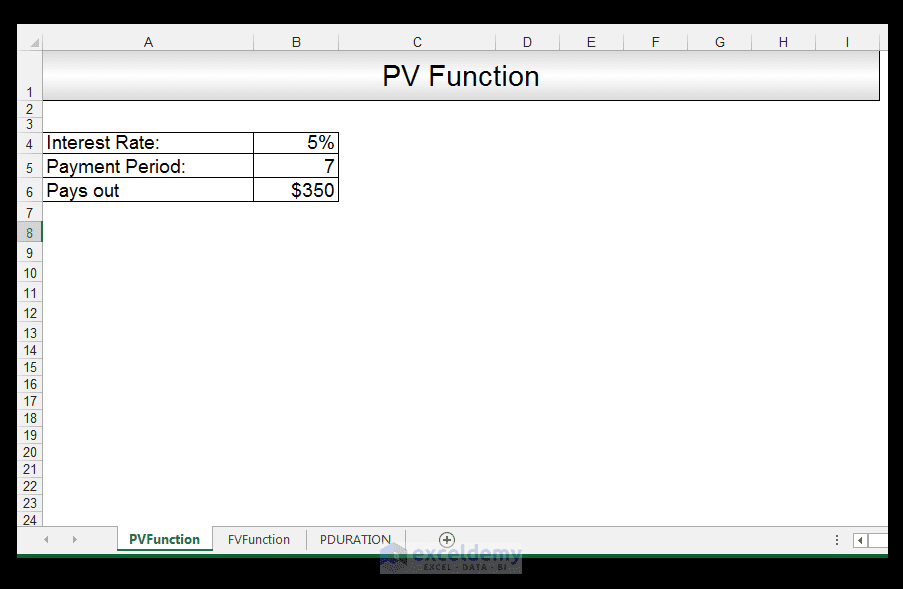1) In order to calculate the PV, in Cell B7 we input the following formula:

=PV (B4/12, B5*12, B6, , 0)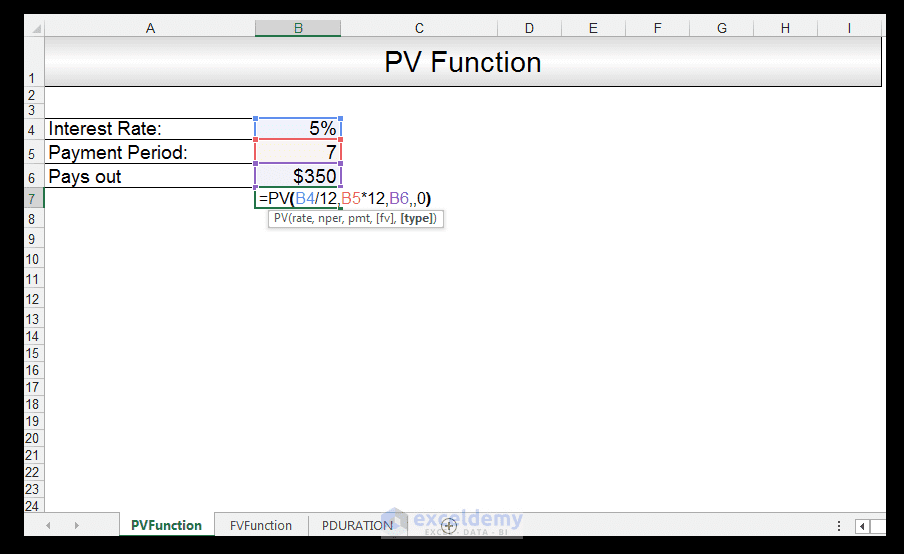We divide the interest rate which is an annual interest rate by 12 in order to get the monthly equivalent and we multiply the 7 years by 12 in order again to convert years to months, this is because payments are monthly.

2) Upon pressing CTRL-ENTER, we get a value of -\$24 763.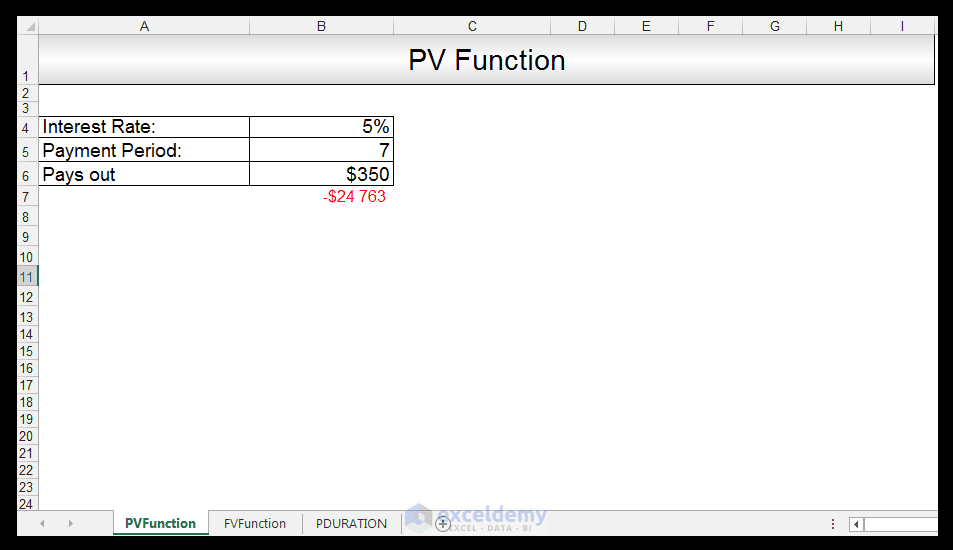3) We expect this initial amount to be negative, since its money paid out of our pockets into the annuity initially.

## How to Use FV Function in Excel (To Find Future Value)

We now want to calculate the future value of an investment, in which we are required to deposit a monthly \$250, over a period of 8 years at an interest rate of 5%.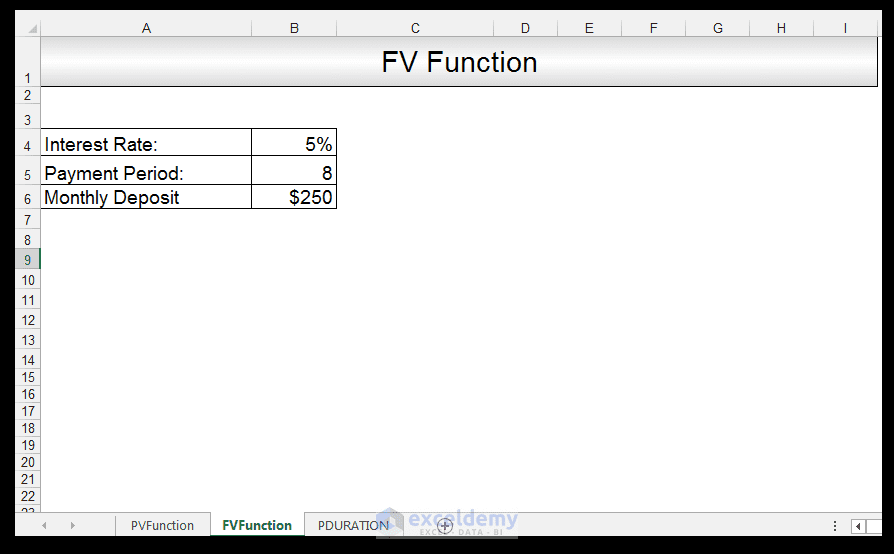1) In order to calculate the FV, in Cell B7 we input the following formula:

=FV (B4/12, B5*12, -250)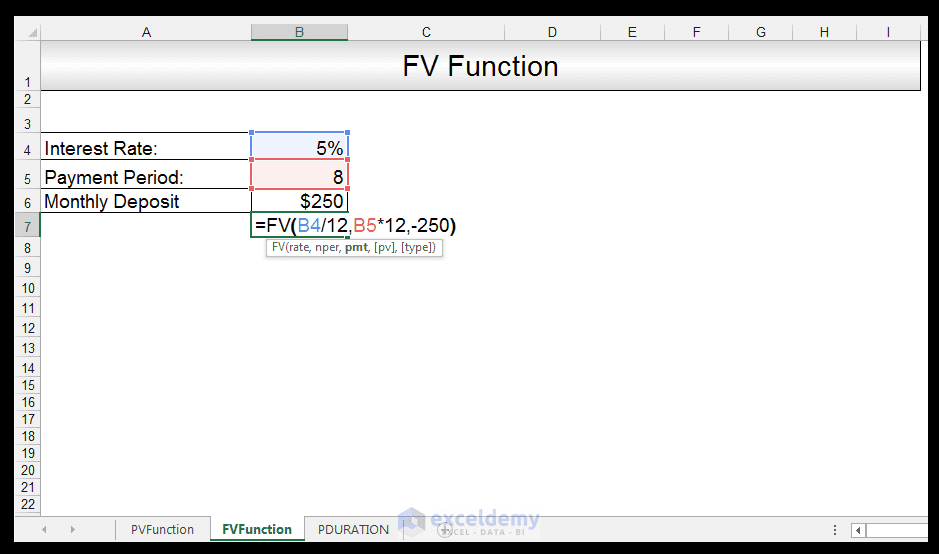Again we divide the interest rate which is an annual interest rate by 12, in order to get the monthly equivalent and we multiply the 8 years by 12, in order again to convert years to months. This is because payments are made monthly, and it’s a negative value of \$250 since we are paying into the investment.

2) Upon pressing CTRL-ENTER, we get a value of \$29 435.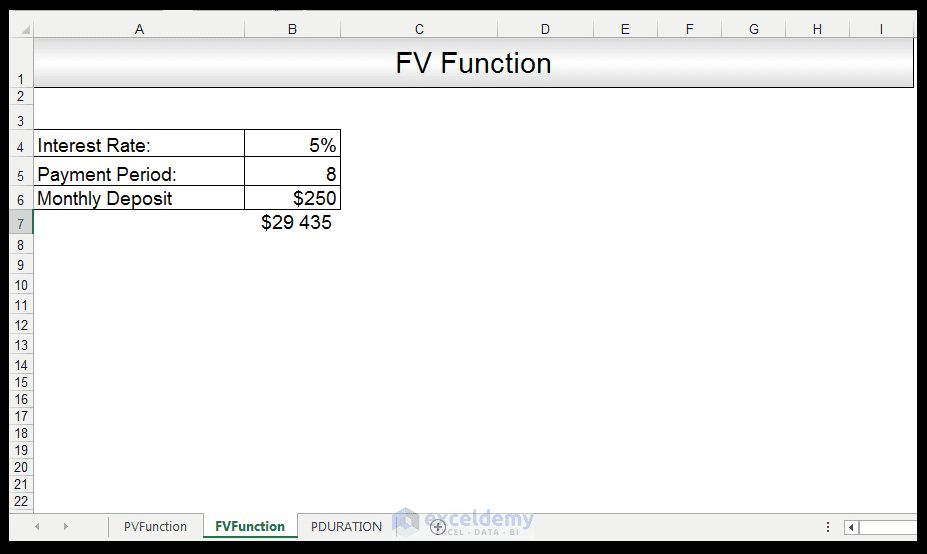3) We expect this future amount to be positive, since its money that will be paid out from the investment into our pockets.

## How to Use Excel PDURATION Function

We want to calculate the number of months, needed by an investment of \$2500, earning 3.7% interest annually, to reach \$3500.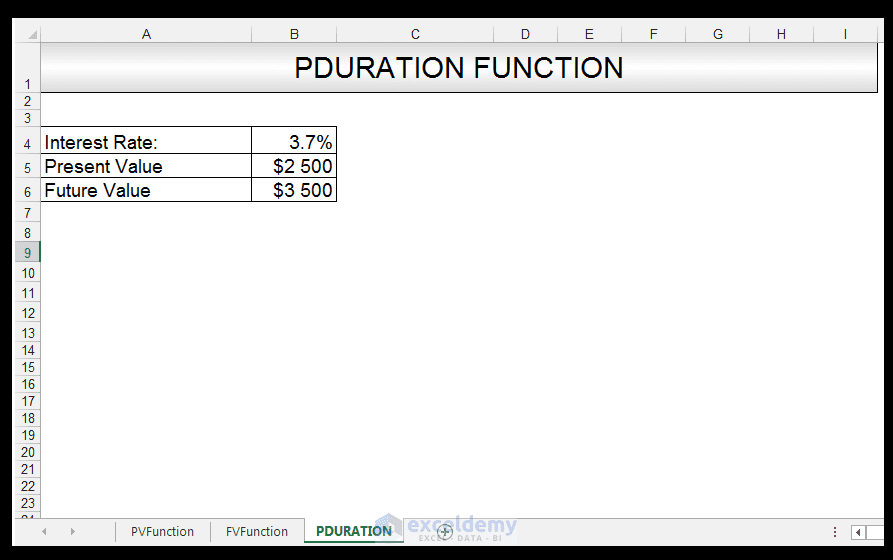1) In order to calculate the PDURATION, in Cell B7 we input the following formula:

=PDURATION (B4/12, B5, B6)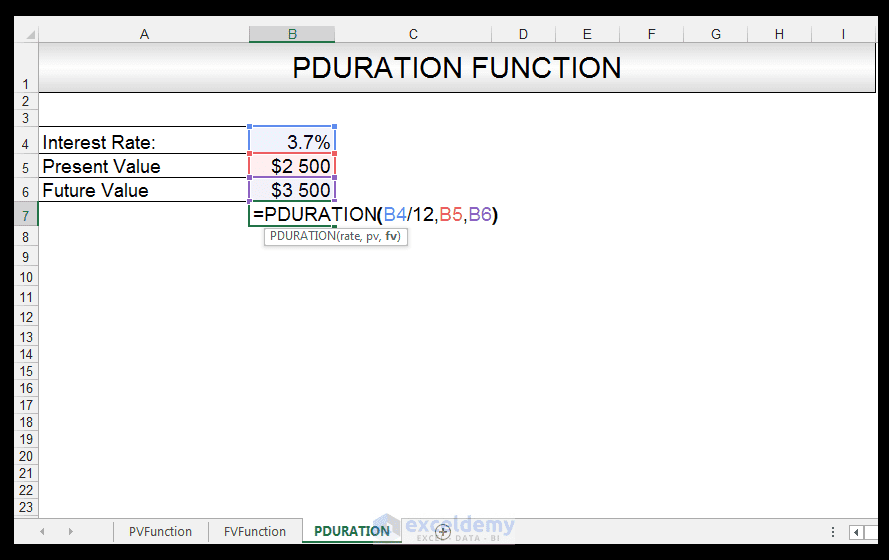Again we divide the interest rate which is an annual interest rate by 12, since we want to ultimately obtain the monthly periods. Note however, that all values for PDURATION are positive.

2) Upon pressing CTRL-ENTER, we get a value of 109.3 months.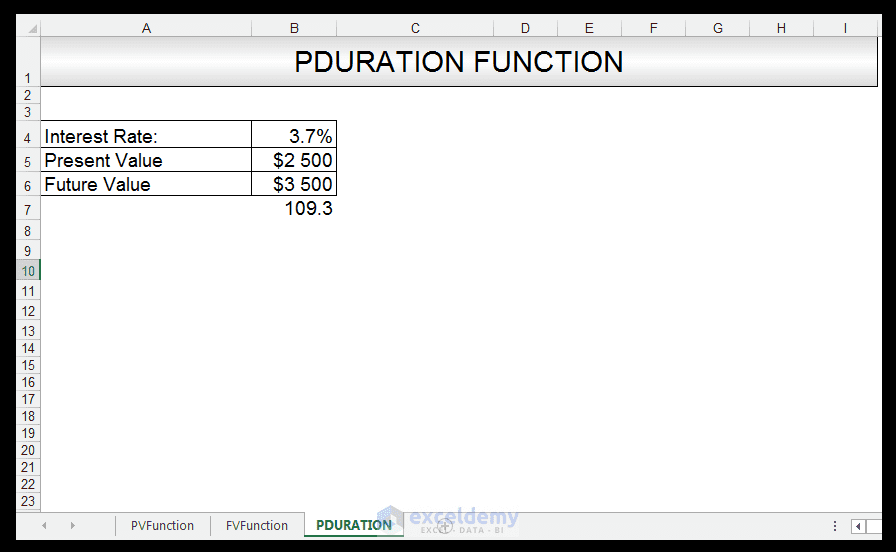3) This value means that it would take 109.3 months, for an investment with an initial value of \$2500 to reach a future value of \$3500.

And there you have it.

PV-FV-PDURATION-Tutorial

## Conclusion

Excel provides many useful financial functions such as Present Value, Future Value, and PDURATION – all of which evaluate inputs at a fixed interest rate.

Please feel free to tell us if you use the PV Function, FV Function or the PDURATION Function in your financial calculations.

Taryn is a Microsoft Certified Professional, who has used Office Applications such as Excel and Access extensively, in her interdisciplinary academic career and work experience. She has a background in biochemistry, Geographical Information Systems (GIS) and biofuels. She enjoys showcasing the functionality of Excel in various disciplines.

1 Comment
1. Replyhaivh May 31, 2017 at 10:26 AM

Dear Ms. Taryn N,
I have a assuming problem in Excel (using PMT function) as below:

“Mr. Adam wants to sign an insurance contract. He expects to earn \$110000 after 18 years at 15% annual interest rate. How much money he must pay monthly for the insurance company, knowing that when signing the contract Mr. Adam paid \$10000?”

rate: 15%/12
nper: 18*12
pv: 10000
fv: 110000

I tried calculate PMT in 4 formulars:
1. =PMT(15%/12,18*12,10000,110000)
2. =PMT(15%/12,18*12,-10000,110000)
3. =PMT(15%/12,18*12,10000,-110000)
4. =PMT(15%/12,18*12,-10000,-110000)
I do not know which formular is right
I’m very confuse in using PMT function, especially using PV and FV parameter sign (- or +).
Could you explain to me the problem above?
I’ve found and read Excel’ help and other examples on the web but still not sure exactly.

Thank you so much and looking forward to hearing from you!